# RD Sharma Solutions Chapter 3 Pair of Linear Equation in Two Variables Exercise 3.3 Class 10 Maths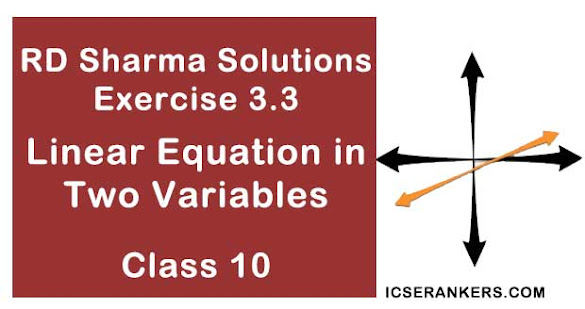Chapter Name RD Sharma Chapter 3 Pair of Linear Equation in Two Variables Book Name RD Sharma Mathematics for Class 10 Other Exercises Exercise 3.1Exercise 3.2Exercise 3.4Exercise 3.5Exercise 3.6 Related Study NCERT Solutions for Class 10 Maths

### Exercise 3.3 Solutions

Solve the following systems of equations:

1. 11x + 15y + 23 = 0
7x – 2y – 20 = 0

Solution

The given system of equation is
11x + 15y + 23 = 0 …(i)
7x – 2y – 20 = 0 …(ii)
From (ii), we get
2y = 7x – 20
⇒ y = (7x – 20)/2
Substituting y = (7x – 20)/2 in (i) we get
11x + 15[(7x – 20)/2] + 23 = 0
⇒ 11x + (105x – 300)/2 + 23 = 0
⇒ (22x + 105x – 300 + 46)/2 = 0
⇒ 127x – 254 = 0
⇒ 127x = 254
⇒ x = 254/127 = 2
Putting x = 2 in y = (7x – 20)/2 we get
⇒ y = (7×2 – 20)/2
= (14 – 20)/2
= -6/2 = -3
Hence, the solution of the given system of equation is x = 2, y = -3.

2. 3x – 7y + 10 = 0
y – 2x – 3 = 0

Solution

The given system of equation is
3x – 7y + 10 = 0 …(i)
y – 2x – 3= 0 …(ii)
From (ii), we get
y = 2x + 3
Substituting y = 2x + 3 in (i) we get
3x – 7(2x + 3) + 10 = 0
⇒ 3x + 14x – 21 + 10 = 0
⇒ - 11x = 11
⇒ x = 11/-11 = -1
Putting x = - 1 in y = 2x + 3, we get
⇒ y = 2×(-1) + 3
= -2 + 3
= 1
⇒ y = 1
Hence, the solution of the given system of equations is x = -1, y = 1.

3. 0.4x + 0.3x = 1.7
0.7x + 0.2y = 0.8

Solution

The given system of equation is
0.4x + 0.3y = 1.7 …(i)
0.7x - 0.2y = 0.8 …(ii)
Multiplying both sides of (i) and (ii), by 10, we get
4x + 3y = 17 …..(iii)
7x – 2y = 8 ….(iv)
From (iv), we get
7x = 8 + 2y
⇒ x = (8 + 2y)/7
Substituting x = (8 +2y)/7 in (iii), we get
4[(8+2y)/7] + 3y = 17
⇒ (32 + 8y)7 + 3y = 17
⇒ 32 + 29y = 17×7
⇒ 29y = 87
⇒ y = 87/29 = 3
Putting y = 3 in x = (8+2y)/7, we get
x = (8 + 2×3)/7
= (8 + 6)/7
= 14/7 = 2
Hence, the solution of the given system of equation is x = 2, y = 3.

4. x/2 +y = 0.8

Solution

x/2 + y = 0.8
And 7/(x+y/2) = 10
∴ x + 2y = 1.6 and (7×2)/(2x + y) = 10
x + 2y = 1.6 and 7 = 10x + 5y
Multiply first equation by 10
10x + 20y = 16 and 10x + 5y = 7
Subtracting the two equations
15y = 9
y = 9/15 = 3/5
x = 1.6 – 2(3/5) = 1.6 – 6/5 = 2/5
Solution is (2/5, 3/5)

5. 7(y+3 ) – 2(x + 3) = 14
4(y – 2) + 3(x – 3) = 2

Solution

The given system of equation is
7(y+3 ) – 2(x + 3) = 14 …(i)
4(y – 2) + 3(x – 3) = 2 …(ii)
From (i), we get
7x + 21 – 2x – 4 = 14
⇒ 7y = 14 + 4 – 21 + 2x
⇒ y = (2x – 3)/7
From (ii), we get
4y – 8 + 3x – 9 = 2
⇒ 4y + 3x – 17 – 2 = 0
⇒ 4y + 3x – 19 = 0 …(iii)
Substituting y = (2x – 3)/7 in (iii), we get
4[(2x – 3)/7] + 3x – 19 = 0
⇒ (8x – 12)/7 + 3x – 19 = 0
⇒ 8x – 12 + 21x – 133 = 0
⇒ 29x – 145 = 0
⇒ 29x = 145
⇒ x = 145/29 = 5
Putting x = 5 in y = (2x – 3)/7, we get
y = (2×5 – 3)/ 7
= 7/7 = 1
⇒ y = 1
Hence, the solution of the given system of equations is x = 5, y = 1.

6. x/7 + y/3 = 5
x/2 – y/9 = 6

Sol: The given system of equation is
x/7 + y/3 = 5 …(i)
x/2 – y/9 = 6 …(ii)
From (i), we get
(3x +7y)/21 = 5
⇒ 3x + 7y = 105
⇒ 3x = 105 – 7y
⇒ x = (105 – 7y)/3
From (ii), we get
(9x – 2y)/18 = 6
⇒ 9x – 2y = 108 ...(iii)
Substituting x = (105 – 7y)/3 in (iii), we get
9[(105 – 7y)/3] – 2y = 108
⇒ (948 – 63y)/3 – 2y = 108
⇒ 945 – 63y – 6y = 108×3
⇒ 945 – 69y = 324
⇒ 945 – 324 = 69y
⇒ 69y = 621
⇒ y = 621/69 = 9
Putting y = 9 in x = (1105 – 7y)/3, we get
x = (105 – 7×9)/3 = (105 – 63)/3
⇒ x = 42/3 = 14
Hence, the solution of the given system of equations is x = 14, y = 9.

7. x/3 + y/4 = 11
5x/6 – y/3 = 7

Solution

The given system of equations is
x/3 + y/4 = 11 …(i)
5x/6 – y/3 = 7 …(ii)
From (i) , we get
(4x + 3y)/12 = 11
⇒ 4x + 3y = 132 …(iii)
From (ii), we get
(5x + 2y)/6 = -7
⇒ 5x – 2y = - 42 …(iv)
Let us eliminate y from the given equations. The coefficients of y in the equations (iii) and (iv) are 3 and 2 respectively. The L.C.M of 3 and 2 is 6. So, we make the coefficient of y equal to 6 in the two equations.
Multiplying (iii) by 2 and (iv) by 3, we get
8x + 6y = 264 …(v)
15x – 6x = -126 …(vi)
Adding (v) and (vi), we get
8x + 15x = 264 – 126
⇒ 23x = 138
⇒ x = 138/23 = 6
Substituting x = 6 in (iii) , we get
4 ×6 + 3y = 132
⇒ 3y = 132 – 24
⇒ 3y = 108
⇒ y = 108/3 = 36
Hence, the solution of the given system of equations is x = 6, y= 36.

8. 4u + 3y = 8
6u – 4y = -5

Solution

Taking 1/x = u, then given equations become
4u + 3y =8 ...(i)
6u – 4y = - 5 …(ii)
From (i) we get
4u = 8 – 3y
⇒ u = (8 – 3y)/4
Substitutig u = (8 – 3y) /4 in (ii), we get
From (ii), we get
6[(8 – 3y)/4] – 4y = -5
⇒ 3(8 – 3y)/2 – 4y = - 5
⇒ (24 – 9y)/2 – 4y = - 5
⇒ (24 – 9y – 8y)/2 = -5
⇒ 24 – 17y = -10
⇒ -17y = -10 – 24
⇒ -17y = -34
⇒ y = -34/-17 = 2
Putting y = 2, in u = (8 – 3y)/4, we get
u = (8 – 3×2)/4 = (8-6)/4= 2/4= ½
Hence, x = 1/u = 2
So, the solution of the given system of equation is x = 2, y = 2.

9. x + y/2 = 4
x/3 + 2y = 5

Solution

The given system of equation is
x + y/2 = 4 …(i)
x/3 + 2y = 5 …(ii)
From (i) , we get
(2x + y)/2 = 4
2x + y = 8
y = 8 – 2x
From (ii), we get
x + 6y = 15 …(iii)
Substituting y = 8 – 2x in (iii), we get
x + 6 (8 – 2x) = 15
⇒ x + 48 – 12x = 15
⇒ -11x = -33
⇒ x = -33/-11 = 3
Putting x = 3, in y = 8 – 2x, we get
y = 8 – 2×3
= 8 – 6 = 2
⇒ y = 2
Hence, solution of the given system of equation is x= 3 , y = 2.

10. x + 2y = 3/2
2x + y = 3/2

Solution

The given system of equation is
x + 2y = 3/2 …(i)
2x + y = 3/2 …(ii)
Let us eliminate y from the given equations. The Coefficients of y in the given equations are 2 and 1 respectively. The L.C.M of 2 and 1 is 2. So, we make the coefficient of y equal to 2 in the two equations.
Multiplying (i) by 1 and (ii) by 2, we get
x + 2y = 3/2 …(iii)
4x + 2y = 3 …(iv)
Subtracting (iii) from (iv), we get
4x – x + 2y – 2y = 3 – 3/2
⇒ 3x = (6 – 3)/2
⇒ 3x = 3/2
⇒ x =3/2×3
⇒ x = ½
Putting x = ½, in equation (iv) , we get
4×1/2 + 2y = 3
⇒ 2 + 2y = 3
⇒ 2y = 3- 2
⇒ y = ½
Hence, solution of the given system of equation is x = 1/2, y = 1/2.

11. √2x + 3y = 0
3x - 8 y = 0

Solution

√2x + √3y = 0 ...(i)
√3x - √8y = 0 …(ii)
From equation (i), we obtain:
x = −√3y/√2 …..(iii)
Substituting this value in equation (ii), we obtain:
√3(−√3y/√2) - √8y = 0
-3y/√2 - 2√2y = 0
y(-3/√2 - 2√2) = 0
y = 0
Substituting the value of y in equation (iii), we obtain:
x = 0
∴ x = 0, y = 0

12. 3x – (y+7)/11 + 2 = 10
2y + (x+ 11)/7 = 10

Solution

The given systems of equation is
3x – (y+7)/11 + 2 = 10 …(i)
2y + (x + 11)/7 = 10 …(ii)
From (i), we get
(33x – y – 7 + 22)/11 = 10
⇒ 33x – y + 15 = 10×11
⇒ 33x + 15 – 110 = y
⇒ y = 33x – 95
From (ii) we get
(14y + x + 11)/7 = 109
⇒ 14y + x + 11 = 10×7
⇒ 14y +x + 11 = 70
⇒ 14y + x = 70 – 11
⇒ 14y + x = 59 …(iii)
Substituting y = 33x – 95 in (iii), we get
14(33x – 95) +x = 59
⇒ 462x – 1330 + x = 59
⇒ 463x = 59 + 1330
⇒ 463x = 1389
⇒ x = 1389/463 = 3
Putting x = 3, in y = 33x – 95 , we get
y = 33×3 – 95
⇒ y = 99 – 95 = 4
⇒ y = 4
Hence, solution of the given system of equation is x = 3, y = 4.

13. 2x – 3/y = 9
3x + 7/y = 2 y
0

Solution

The given system of equation is
2x – 3/y = 9 …(i)
3x + 7/y = 2, y ≠ 0 …(ii)
Taking 1 /y = u, the given equations becomes
2x – 3u = 9 …(iii)
3x + 7u = 2 …(iv)
From (iii), we get
2x = 9 + 3u
⇒ x = (9 + 3u)/2
Substituting x = (9+3u)/2 in (iv) , we get
3(9 +3u)/2 + 7u = 2
⇒ (27+ 9u + 14u)/2 = 2
⇒ 27 + 23u = 2×2
⇒ 23u = 4 – 27
⇒ u = -23/23 = - 1
Hence, y = 1/u = 1/-1 = - 1
Putting u = -1 in x = (9+ 3u)/2, we get
x = (9 + 3×-1)/2 = (9 -3)/2 = 6/2 = 3
⇒ x = 3
Hence, solution of the given system of equation is x = 3, y = -1.

14. 0.5x + 0.7y = 0.74
0.3x + 0.5y = 0.5

Sol : The given systems of equations is
0.5x + 0.7y = 0.74 …(i)
0.3x + 0.5y = 0.5 …(ii)
Multiplying (i) and (ii) by 100, we get
50x + 70y = 74 …(iii)
30x + 50y = 50 …(iv)
From (iii), we get
50x = 74 – 70y
x = (74 – 70y)/50
Substituting x = (74 – 70y)/50 in equation (iv), we get
30(74 – 70y)/50 + 50y = 50
⇒ 3(74 – 70y)/5 + 50y = 50
⇒ (222 – 210y)/5 + 50y = 50
⇒ 222 – 210y + 250y = 250
⇒ 40y = 250 – 222
⇒ 40y = 28
⇒ y = 28/40 = 14/20 = 7/10 = 0.7
Putting y = 0.7 in x = (74 – 70y)/50 , we get
x = (74 – 70×0.7)/50
= (74 – 49 )/50
= 25/50 = 1/2 = 0.5
Hence, solution of the given system of equation is x = 0.5, y = 0.7

15. 1/7x + 1/6y = 3
1/2x – 1/3y = 5

Solution

1/7x + 1/6y = 3 …(i)
1/2x – 1/3y = 5 …(ii)
Multiplying (ii) by 1/2, we get
1/7x + 1/6y = 3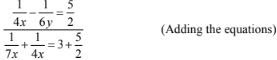⇒ (4+7)/28x = (6+5)/2
⇒ 11/28x = 11/2
⇒ x = (11×2)/(28×11) = 1/14
When x = 1/14, we get
1/7(1/14) + 1/6y = 3 (Using (i)]
⇒ 2 + 1/6y = 3
⇒ 1/6y = 3 – 2 = 1
⇒ y = 1/6
Thus , the solution of given equation is x = 1/14 and y = 1/6.

16. 1/2x + 1/3y = 2
1/3x + 1/2y = 13/6

Solution

Let 1/x = u and 1/y = v, the given equations become
u/2 + v/3 = 2
⇒ (3u + 2v)/6 = 2
⇒ 3u + 2v = 12 …(i)
And, u/3 + v/2 = 13/6
⇒ (2u + 3v)/6 = 13/6
⇒ v = 6/2 = 3
Hence, x = 1/u = 1/2 and y = 1/v = 1/3
So, the solution of the given system equation is x = 1/2, y = 1/3.

17. (x +y)/xy = 2
(x – y)/xy = 6

Solution

The given system of equation is
(x + y)/xy = 2
⇒ x/xy + y/xy = 2
⇒ 1/y + 1/x = 2 ….(i)
And, (x – y)/xy = 6
⇒ x/xy – y/xy = 6
⇒ 1/y – 1/x = 6 ….(ii)
Taking 1/y = v and 1/x = u, the above equations become
v + u = 2 ….(iii)
v – u = 6 ….(iv)
Adding equation (iii) and equation (iv) , we get
v+ u + v – u = 2 + 6
⇒ 2v = 8
⇒ v = 8/2 = 4
Putting v = 4 in equation (iii), we get
4 + u = 2
⇒ u = 2 – 4 = - 2
Hence, x = 1/u = 1/ - 2 = -1/2 and y = 1/v = 1/4
So, the solution of the given system of equation is x = -1/2, y = 1/4

18. 15/u + 2/v = 17

Solution

Let 1/u = x and 1/v = y, then the given system of equations become
15x + 2y = 17 ….(i)
x + y = 36/5 …(ii)

From (i), we get
2y = 17 – 5x
⇒ y = (17 – 15x)/2
Substituting y = (17 – 15x)/2 in equation (ii), we get
x + (17 – 15x)/2 = 36/5
⇒ (2x + 17 – 15x)/2 = 36/5
⇒ (-13x + 17)/2 = 36/5
⇒ 5(-13x + 17) = 36×2
⇒ -65x + 85 = 72
⇒ -65x = 72 – 85
⇒ -65x = -13
⇒ x = -13/-65 = 1/5
Putting x = 1/5 in equation (ii), we get
1/5 + y = 36/5
⇒ y = 36/5 – 1/5
⇒ y = 35/5 = 7
Hence u = 1/x = 5 and v = 1/y = 1/7 .
So, the solution of the given system of equation is u = 5, v = 1/7.

19. 3/x – 1/y = - 9
2/x + 3/y = 5

Solution

Let 1/x = u and 1/y = v, Then, the given system of equations becomes
3u – v = -9 ...(i)
2u + 3v = 5 …(ii)
Multiplying equation (i) by 3 and equation (ii) by 1, we get
9u – 3v = -27 …(iii)
2u + 3v = 5 …(iv)
Adding equation (i) and equation (ii), we get
9u + 2u – 3v + 3v = -27 + 5
⇒ 11u = -22
⇒ u = -22/11 = -2
Putting u = -2 in equation (iv), we get
2×(-2) + 3v = 5
⇒ -4 + 3v = 5
⇒ 3v = 5 + 4
⇒ v = 9/3 = 3
Hence, x = 1/u = 1/-2 = -1/2 and y = 1/v = 1/3.
So, the solution of the given system of equation is x = -1/2, y = 1/3.

20. 2/x + 5/y = 1
60/x + 40y = 19, x
0, y 0

Solution

Taking 1/x = u and 1/y = v, the given becomes
2u + 5v = 1 …(i)
60u + 40u = 19 …(ii)
Let us eliminate ‘u’ from equation (i) and (ii), multiplying equation (i) by 60 and equation (ii) by 2, we get
120u +300v = 60 …(iii)
120u + 80v = 38 …(iv)
Subtracting (iv) from (iii), we get
300v – 80v = 60 – 38
⇒ 220v = 22
⇒ v = 22/220 = 1/10
Putting v = 1/10 in equation (i), we get
2u + 5×1/10 = 1
⇒ 2u + 1/2 = 1
⇒ 2u = 1 – 1/2
⇒ 2u = (2-1)/2 = 1/2
⇒ 2u = 1/2
⇒ u = 1/4
Hence, x = 1/u = 4 and y = 1/v = 10
So, the solution of the given system of equation is x = 4 , y = 10.

21. 1/5x + 1/6x = 12
1/3x – 3/7y = 8, x
0, y0

Solution

Taking 1/x = u and 1/y = v, the given equations become
u/5 + v/6 = 12
⇒ (6u + 5v)/30 = 12
⇒ 6u + 5v = 360 …(i)
And u/3 – 3v/7 = 8
⇒ (7u + 9v)/21 = 8
⇒ 7u – 9v = 168 …(ii)
Let us eliminate ‘v’ from equation (i) and (ii), multiplying equation (i) by 9 and equation (ii) by 5, we get
54u + 45v = 3240 …(iii)
35u – 45v = 840 …(iv)
54u + 35u = 3240 + 840
⇒ 89u = 4080
⇒ u = 4080/89
Putting u = 4080/89 in equation (i) , we get
6× 4080/89 + 5v = 360
⇒ 24480/89 + 5v = 360
⇒ 5v = 360 – 24480/89
⇒ 5v = (32040- 24480)/89
⇒ 5v = 7560/89
⇒ v = 7560/5×89
⇒ v = 1512/89
Hence, x = 1/u = 89/4080 and y = 1/v = 89/1512
So, the solution of the given system of equation is x = 89/4080, y = 89/1512

22. 2/x + 3/y = 9/xy
4/x + 9/y = 21/xy , where x
0, y  0

Solution

The system of given equation is
2/x + 3/y = 9/xy …(i)
4/x + 9/y = 21/xy , where x ≠0, y = 0 …(ii)
Multiplying equation (i) adding equation (ii) by xy, we get
2y + 3x = 9 …(iii)
4y + 9x = 21 …(iv)
From (iii), we get
3x = 9 – 2y
⇒ x = (9 – 2y)/3
Substituting x = (9 – 2y)/3 in equation (iv) , we get
4x + 9(9-2y)/3 = 21
⇒ 4y + 3(9 -2y) = 21
⇒ 4y + 27 – 6y = 21
⇒ -2y = 21 – 27
⇒ -2y = -6
⇒ y =3
Putting y = 3 in x = (9-2y)/3 , we get
x = (9 – 2×3)/3
= (9-6)/3
= 3/3 = 1
Hence, solution of the system of equation is x = 1, y = 3

23. 6/(x+y) = 7/(x – y) + 3
1/2(x+ y) = 1/3(x – y), where x + y
0 and x – y  0

Solution

Let 1/x + y = u and 1/x – y = v. Then the given system of equation becomes
6u = 7v + 3
⇒ 6u – 7v = 3 …(i)
And, u/2 =v/3
⇒ 3u = 2v
⇒ 3u – 2v = 0 …(ii)
Multiplying equation (ii) by 2, and equation (i) by 1, we get
6u – 7v = 3 …(iii)
6u – 4v = 0 …(iv)
Subtracting equation (iv) from equation (iii), we get
- 7 + 4v = 3
⇒ -3v = 3
⇒ v = -1
Putting v = -1 in equation (ii), we get
3u – 2×(-1) = 0
⇒ 3u + 2 = 0
⇒ 3u = -2
⇒ u = -2/3
Now, u = -2/3
⇒ 1/(x +2) = -2/3
⇒ x + y = -3/2 …(v)
And, v = - 1
⇒ 1/(x –y) = -1
⇒ x –y = -1 …(vi)
Adding equation (v) and equation (vi), we get
2x = -3/2 – 1
⇒ 2x = (-3-2)/2
⇒ 2x = -5/2
⇒ x =-5/4
Putting x = -5/4 in equation (vi) , we get
-5/4 – y = -1
⇒ -5/4 + 1 = y
⇒ (-5+4)/4 = y
⇒ -1/4 = y
⇒ y = -1/4
Hence, solution of the system of equation is x = -5/4, y = -1/4.

24. xy/(x+y) = 6/5
xy/(y-x) = 6

Solution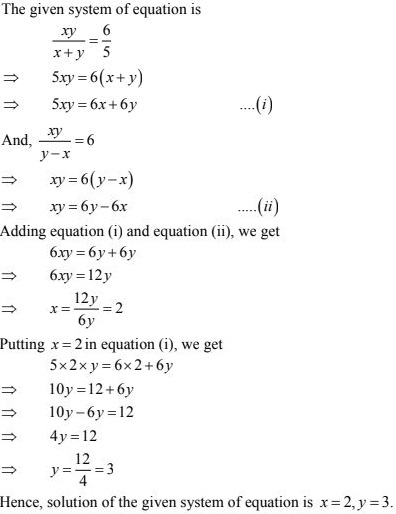25. 22/(x+y) + 15/(x -y) = 5
55/(x +y)  + 45/(x -y) = 14

Solution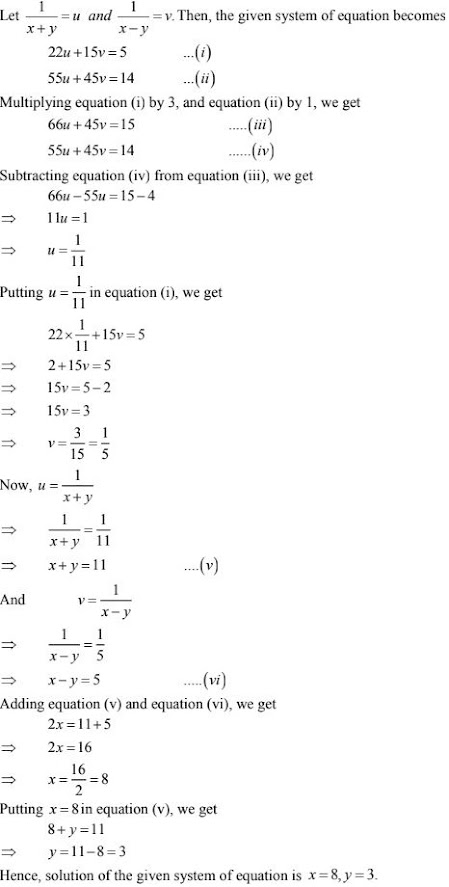26. 5/(x+y) - 2/(x-y) = -1
15/(x+ y) + 7/(x -y) = 10

Solution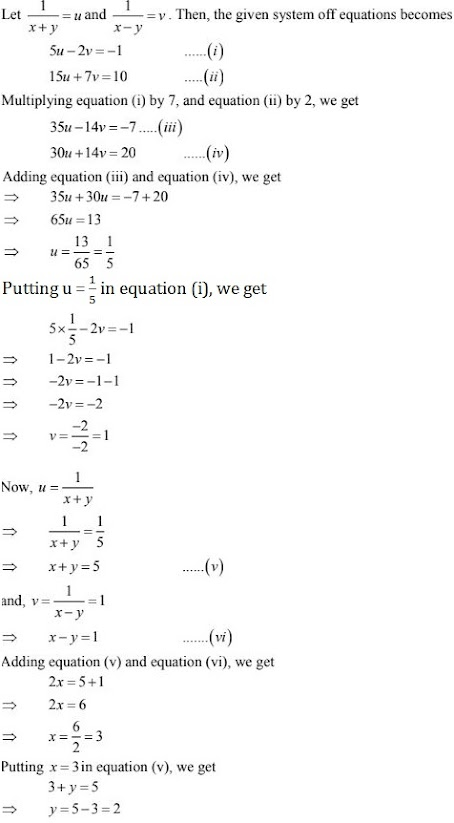Hence, solution of the given system of equation is x = 3, y = 2 .

27. 3/(x+y) + 2/(x-y) = 2
9/(x+y) - 4/(x-y) = 1

Solution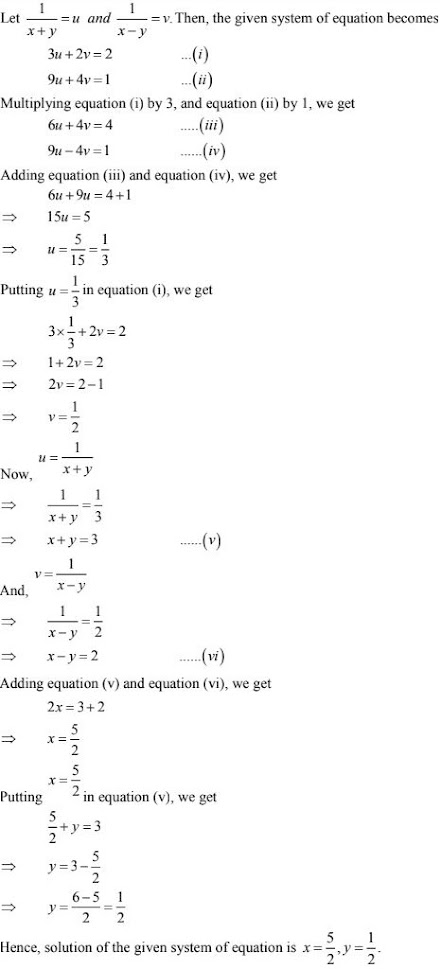28. 1/2(x+2y) + 5/3(3x-2y) = -3/2
5/4(x+2y) - 3/5(3x-2y) = 61/60

Solution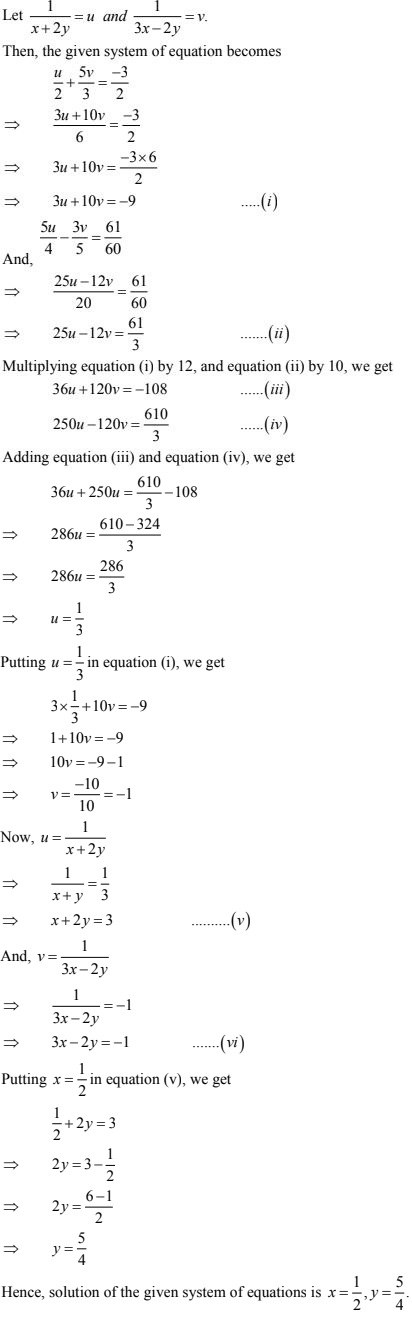29. 5/(x+1) - 2/(y-1) = 1/2
10/(x+1) + 2/(y-1) = 5/2,  where x ≠ -1 and y ≠ 1

Solution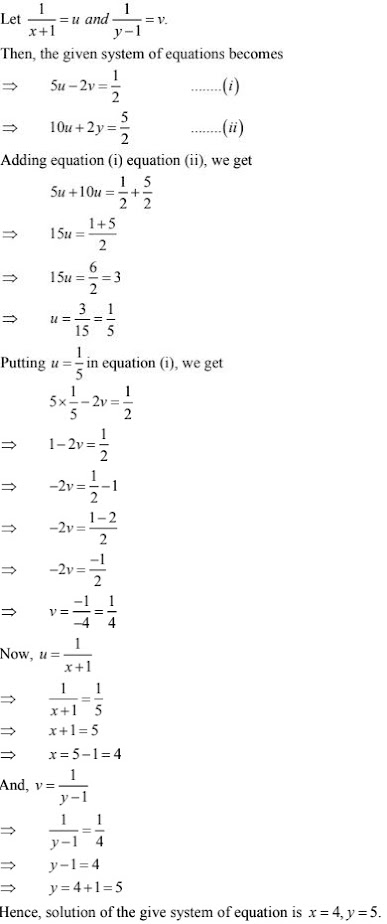30. x+y = 5xy
3x + 2y = 13xy

Solution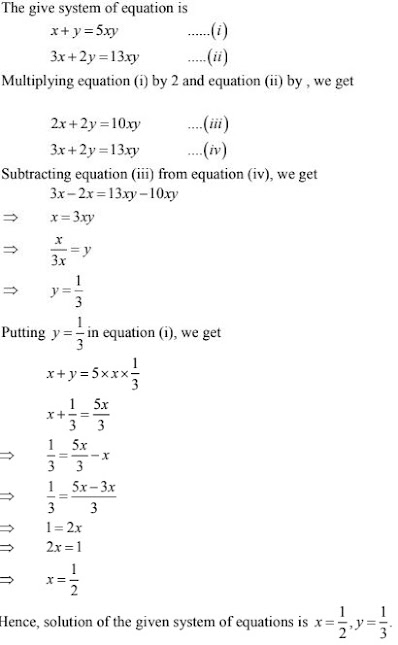31. x+ y = 2xy
(x-y)/xy = 6 x ≠ 0,  y ≠ 0

Solution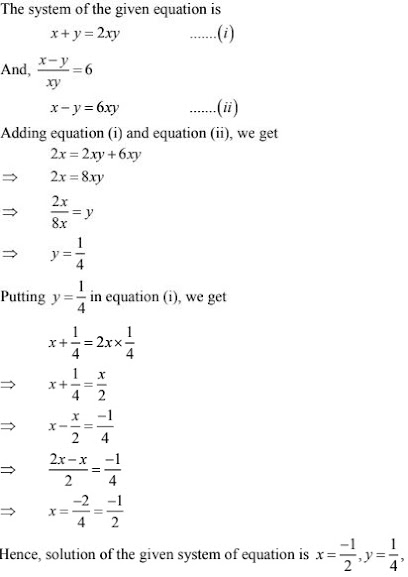32. 2(3u - v) = 5uv
2(u + 3v) = 5uv

Solution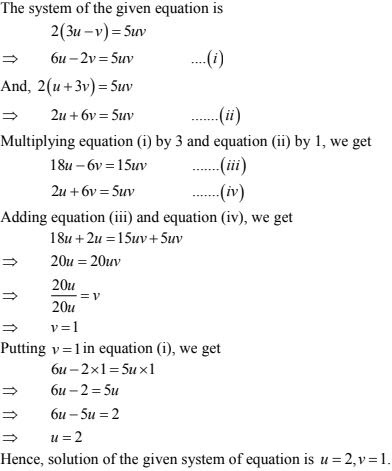33. 2/(3x + 2y) + 3/(3x - 2y) = 17/5
5/(3x + 2y) + 1/(3x -2y) = 2

Solution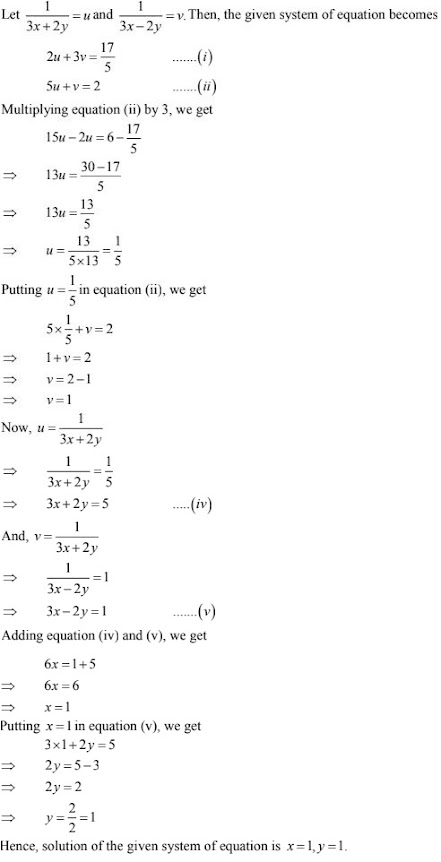34. 4/x + 3y = 14
3/x -4y = 23

Solution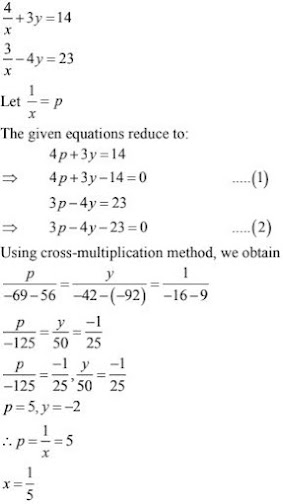35. 99x + 101y = 499
101x + 99y = 501

Solution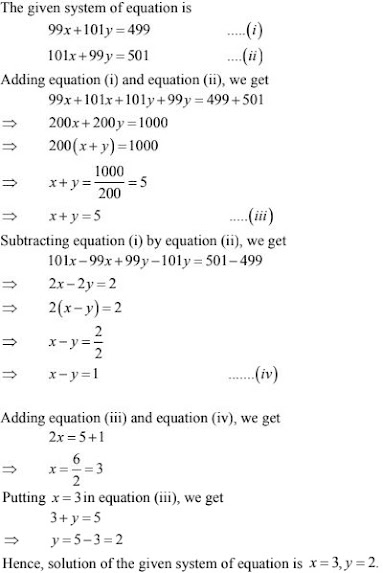36. 23x - 29y = 98
29x - 23y = 110

Solution

The given system of equation is
23x - 29y = 98 ...(i)
29x - 23y = 110 ...(ii)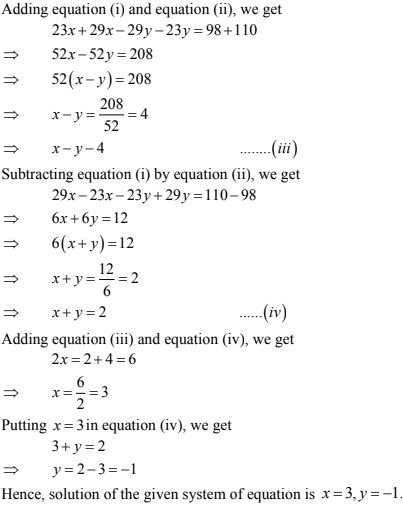37. x - y +z = 4
x - 2y -2z = 9
2x + y + 3z = 1

Solution

We have,
x - y +z = 4 ...(i)
x - 2y -2z = 9 ...(ii)
2x + y + 3z = 1 ...(iii)
From equation (i) , we get
z = 4 - x + y
⇒ z = -x + y + 4
Subtracting the value of z in equation (ii), we get38. x - y +z = 4
x + y + z = 2
2x + y  - 3z = 0

Solution

We have,
x - y +z = 4 ...(i)
x + y + z = 2 ...(ii)
2x + y  - 3z = 0 ...(iii)
From equation (i), we get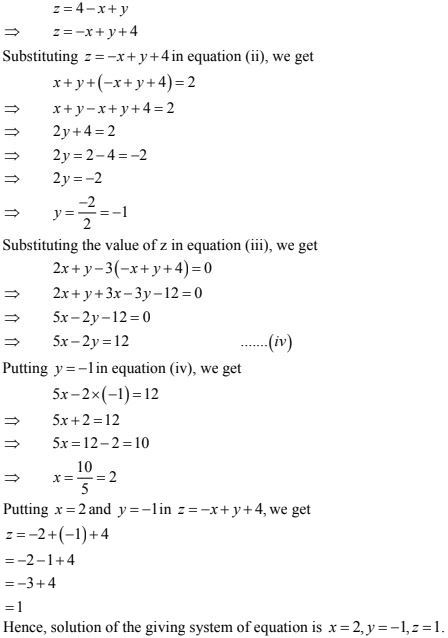39. 44/(x+y) + 30/(x-y) = 4
55/(x+y) + 40/(x-y) = 13

Solution

Let 1/(x+ y) = u and 1/(x -y) = v.
Then, the system of the given equations becomes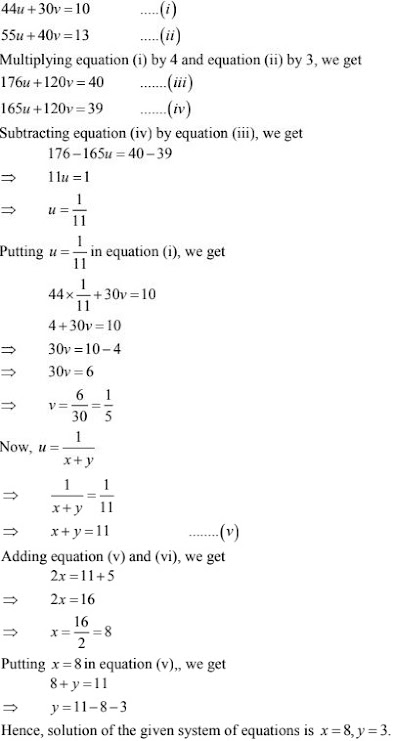40. 4/x + 15y = 21
3/x + 4y = 5

Solution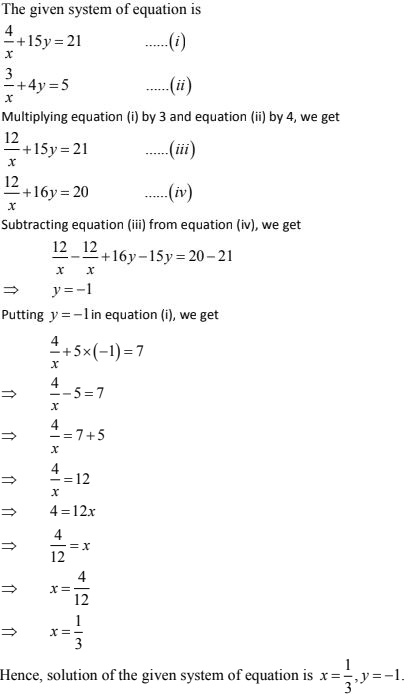41. 2(1/x) + 3(1/y) = 13
5(1/x) - 4(1/y) = -2

Solution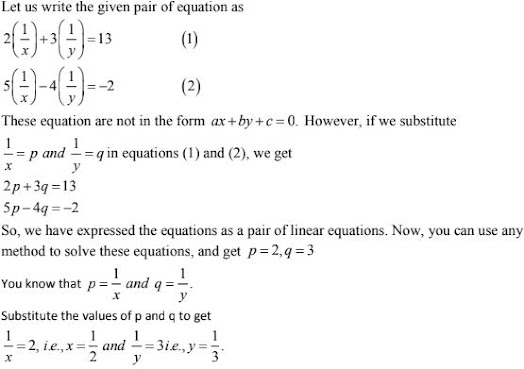42. 5/(x-1) + 1/(y-2) = 2
6/(x-1) - 3/(y-2) = 1

Solution

5/(x-1) + 1/(y-2) = 2 ...(i)
6/(x-1) - 3/(y-2) = 1 ...(ii)
Let 1/(x - 1) = u, 1/(y-2) = v
So, our equations become
5u+ v = 2 ...(iii)
6u - 3v = 1 ...(iv)
From equation (iii),
5u +  v = 2
v = 2 - 5u ...(v)
Putting value of v in (iv)
6u - 3v = 1
6u - 3(2 - 5u) = 1
6u - 6 + 15u = 1
21u = 1+6
u = 7/21 = 1/3
Putting u = 1/3 in equation (v)
v = 2 - 5u
= 2 - 5×1/3
= 2 - 5/3
= (6-5)/3 = 1/3
Now, 1/(x-1) = u
1/(x -1) = 1/3
⇒ x -1 = 3
⇒ x = 4
and , 1/(y - 2) = v
⇒ 1/(y-2) = 1/3
⇒ y - 2 = 3
⇒ y = 5
Hence, solution of the given system of equation is x = 4, y = 5.

43. 10/(x+y) + 2/(x-y) = 4
15/(x+y) - 5/(x-y) = -2

Solution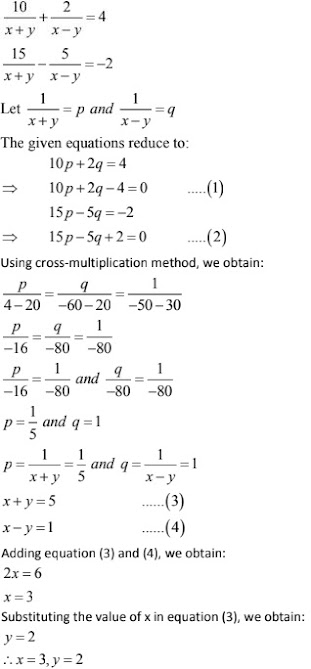44. 1/(3x+y)+ 1/(3x-y) = 3/4
1/2(3x+y) - 1/2(3x- y) = -1/8

Solution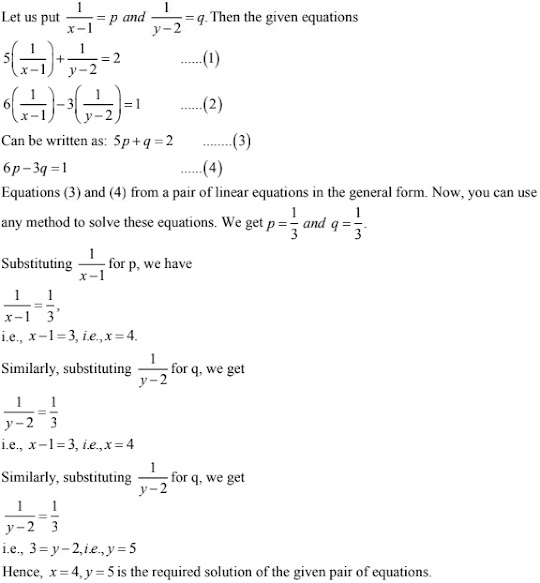45. 2/√x + 3/√y = 2
4/√x - 9/√y = -1

Solution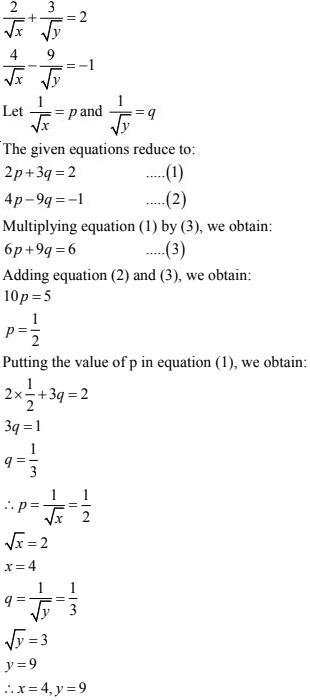46. (7x - 2y)/xy = 5
(8x + 7y)/xy = 15

Solution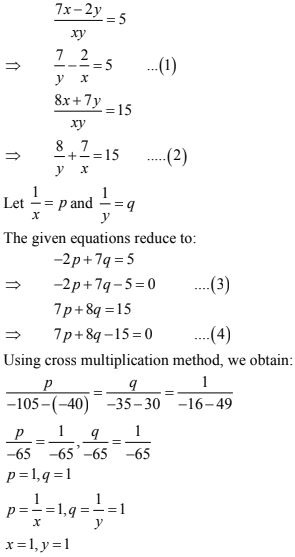47. 152x - 378y = -74
-378x + 152y = -604

Solution

152x - 378y = -74 ...(i)
-378x + 152y = -604 ...(ii)
Adding the equations (i) and (ii), we obtain:
-226x - 226y = -678
⇒  x + y = 3 ...(iii)
Subtracting the equation(ii) from equation (i) , we obtain
530x - 530y = 530
⇒ x - y = 1 ...(iv)
Adding equations (iii) and (iv), we obtain:
2x = 4
x = 2
Substituting the value of x in equation (iii) , we obtain:
y = 1